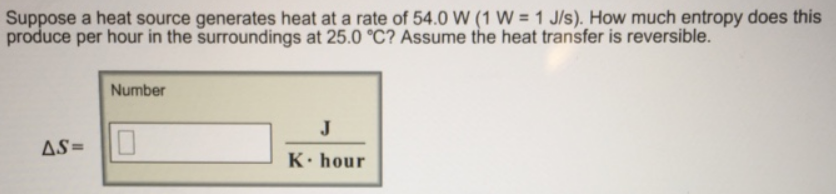# Problem: Suppose a heat source generates heat at a rate of 54.0 W (1 W = 1 J/s). How much entropy does this produce per hour in the surroundings at 25.0 °C? Assume the heat transfer is reversible.

###### FREE Expert Solution
83% (384 ratings)###### Problem Details

Suppose a heat source generates heat at a rate of 54.0 W (1 W = 1 J/s). How much entropy does this produce per hour in the surroundings at 25.0 °C? Assume the heat transfer is reversible.